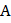# Two rods of same material and length have their electric resistances in ratio 1 : 2.  When both rods are dipped in water, the correct statement will be a)has more loss of weight b)has more loss of weight c) Both have same loss of weight d) Loss of weight will be in the ratio 1 : 2

## Question ID - 152014 :- Two rods of same material and length have their electric resistances in ratio 1 : 2.  When both rods are dipped in water, the correct statement will be a)has more loss of weight b)has more loss of weight c) Both have same loss of weight d) Loss of weight will be in the ratio 1 : 2

3537

 (a)Now, when a body dipped in water, loss of weightSo,;sohas more loss of weight
 Three resistances each ofare joined in parallel. Three such combinations are put in series, then the resultant resistance will be a)b)c)d)